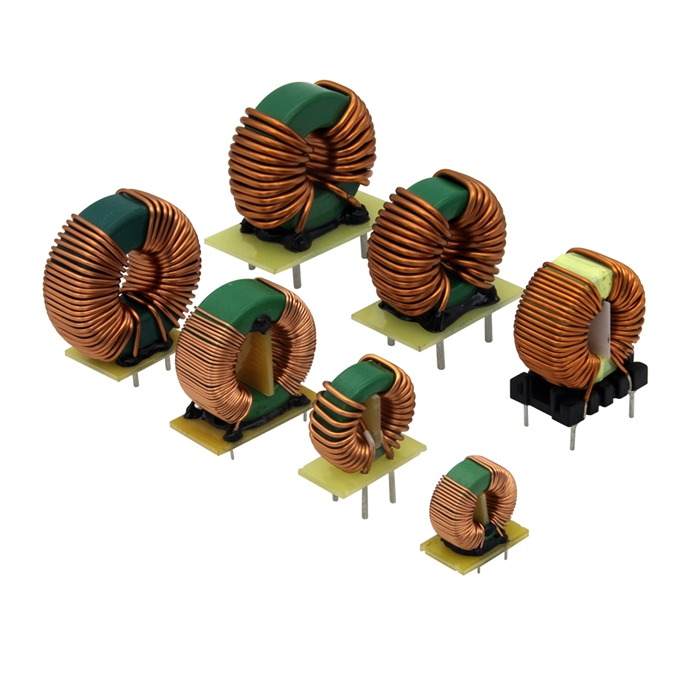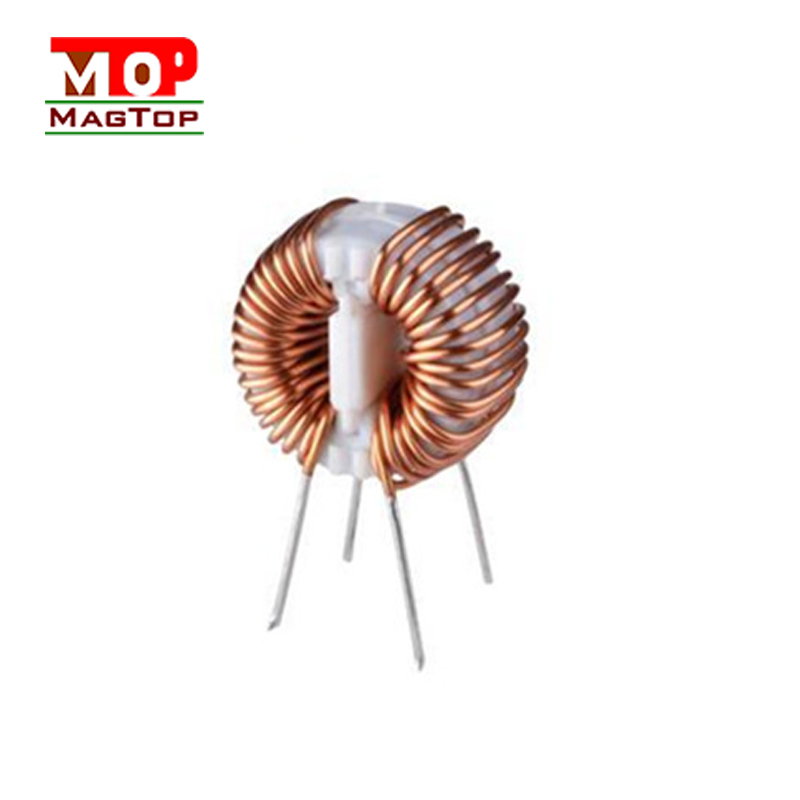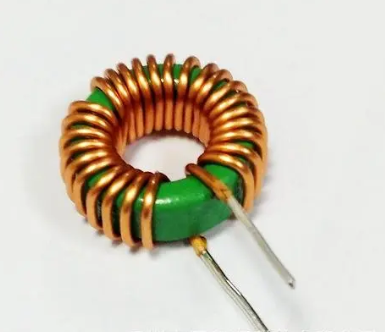# Difference Between Common Mode Inductor and Differential Mode Inductor

##### author: MagTop
08/08/2022Common mode inductors and differential mode inductors are commonly used filter inductors in circuits. The two often exist in the form of toroidal inductor coils. There are many similarities in appearance. Today, I will introduce the difference between common mode inductors and differential mode inductors. .

First of all, we distinguish from the concept, mainly divided into the following five points:

1. The differential mode interference signal is a current loop signal formed in opposite directions between the two input power lines, and the common mode signal is a current loop signal formed by the two input lines in the same direction and the ground.
2. To put it simply, differential mode means that the signals on the two trunks of the transmission loop between the circuits are opposite, and the common mode means that the signals on the two trunks have the same phase.
3. Common mode inductors are generally wound in two directions; differential mode inductors are wound in one direction.
4. The filter inductor that suppresses common mode interference is called common mode inductor; the filter inductor that suppresses differential mode interference is called differential mode inductor.
5. The number of turns wound on the same core is equal, the diameter of the wire is the same, and the two sets of coils with opposite windings are common mode inductance; differential mode inductance is a coil wound on one core.

Secondly, from the appearance point of view, you can find the most obvious difference between the two: the common mode inductor has 4 pins, and the differential mode inductor has 2 pins. For details, you can refer to the following figure:

According to the above method, it will be much easier for us to judge, but before using this method, there are some details that we cannot ignore:

1. The common mode inductor is two windings, with the same input and the same output, and the end with the same name is generally located on the same side of the magnetic ring
2. The number of turns of the common mode inductor is the same. If the distribution is asymmetric, it is not a common mode inductor.

3. If the wiring is wrong, the common mode inductance will be effective.

Now, let's summarize the characteristics of common mode inductors and differential mode inductors separately to help you better distinguish them.

The characteristics of common mode inductance: Since the two sets of coils on the same iron core are wound in opposite directions, the iron core is not afraid of saturation. High conductivity ferrite material is the most used magnetic core material on the market.

The characteristics of differential mode inductors: applied in high current applications, due to the coil wound in an iron core manufacturer, when the current flowing into the coil increases, the iron core of the coil will be saturated, and the iron powder core material is the most popular in the market. The most used core material is mainly because the price is relatively cheap.